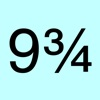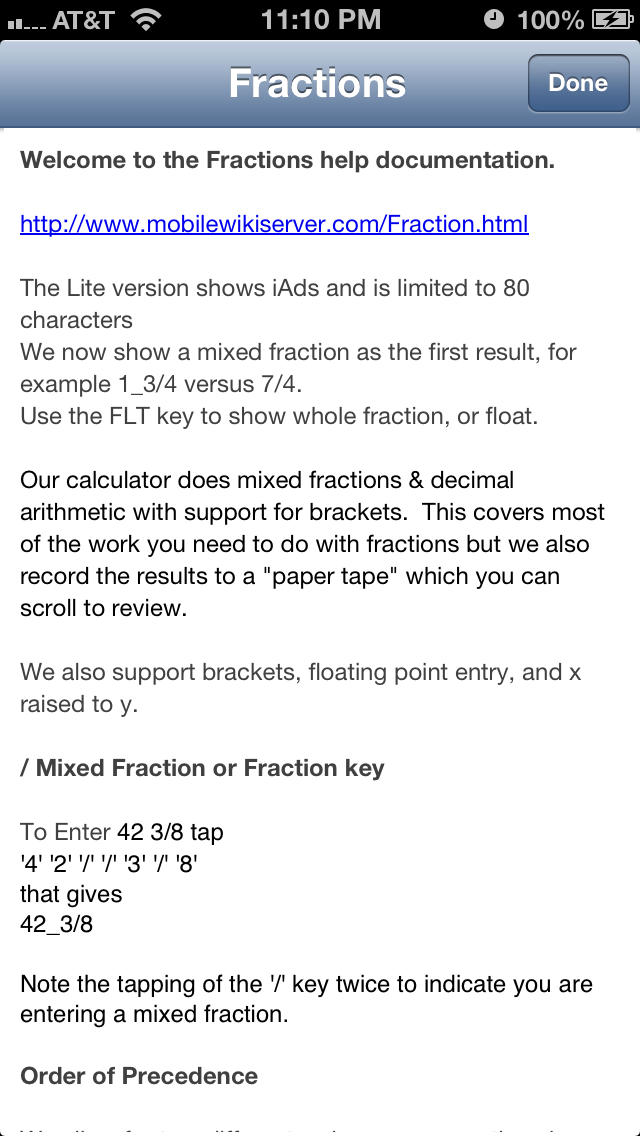# Fractions Calculator Lite - Lumos Educational App Store41
Price - Free
\$0

#### DESCRIPTION:

5 3/16 (12.11 - 1/8) 2.0 = to get the answer 29 63/400 which you can then show as11663/400, or 29.1575Fractions calculator is a fraction calculator that does mixed or whole fractions & decimal arithmetic with support for brackets

#### OVERVIEW:

Fractions Calculator Lite is a free educational mobile app By Corporate Smalltalk Consulting Ltd.It helps students in grades 3,4,5 practice the following standards 3.NF.1,4.NF.1,5.NF.1.

This page not only allows students and teachers download Fractions Calculator Lite but also find engaging Sample Questions, Videos, Pins, Worksheets, Books related to the following topics.

1. 3.NF.1 : Understand a fraction 1/b as the quantity formed by 1 part when a whole is partitioned into b equal parts; understand a fraction a/b as the quantity formed by a parts of size 1/b. (Grade 3 expectations in this domain are limited to fractions with denominators 2, 3, 4, 6, and 8.).

2. 4.NF.1 : Explain why a fraction a/b is equivalent to a fraction (n .

3. 5.NF.1 : Add and subtract fractions with unlike denominators (including mixed numbers) by replacing given fractions with equivalent fractions in such a way as to produce an equivalent sum or difference of fractions with like denominators. For example, 2/3 + 5/4 = 8/12 + 15/12 = 23/12. (In general, a/b + c/d = (ad + bc)/bd.) .

3
4
5

#### STANDARDS:

3.NF.1
4.NF.1
5.NF.1

Developer: Corporate Smalltalk Consulting Ltd

Software Version: 1.4

Category: Utilities

Release Date: 2010-07-06T17:32:31Z### RELATED APPSEdSearch WebSearch